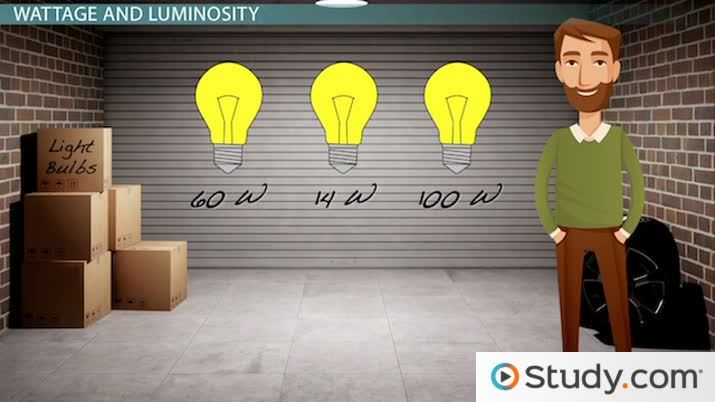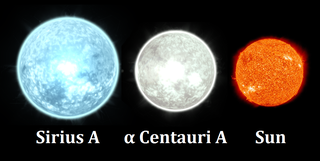# Luminosity of a Star: Measurement & Formulas

Kelly Barkers, Artem Cheprasov
• Author
Kelly Barkers

Kelly taught 7th grade science for 5 years and served as the Science Department Chair. She graduated from Cedar Crest College with a bachelor's degree in biology and a minor in health and wellness. While attending college, she was a peer tutor for Anatomy and Physiology.

• Instructor
Artem Cheprasov

Artem has a doctor of veterinary medicine degree.

Learn about the luminosity of a star. Discover the difference between apparent brightness and luminosity and understand the luminosity formula, with examples. Updated: 12/26/2021

Show

## What is Luminosity?

Professional astronomers, amateurs or anyone who has looked up in the nighttime sky has probably noticed the star Sirius. Located in the constellation Canis Major, Sirius is the brightest star visible on Earth, aside from the Sun. However, this does not mean that Sirius generates the most energy nor is the largest or hottest star. Compared to Earth's Sun, Sirius is only 1.7 times larger in radius. On the other hand, Rigel, a star in the Orion constellation, is nearly 80 times larger in radius than Earth's Sun but is only the 7th brightest star in the nighttime sky. How is one star so much smaller but able to shine so much brighter? The answer lies in perception.

The brightness of an object is the quality of the light that appears to shine from it and is influenced by the observer's distance to the object, For example, Sirius is only located 8.6 light years away from Earth while Rigel is quite a bit father at 863 light years away. Therefore, Rigel appears to be more dim because of the greater distance between the star and Earth. In order to accurately measure the amount of light from a star, scientists developed an equation that measures luminosity. Luminosity is the intrinsic or actual amount of light given off by an object. Specifically, luminosity in the total amount of electromagnetic energy released by a star per unit of time. Rigel's luminosity is 120,000 times brighter than the Sun while Sirius' luminosity is only about 25 times brighter than the Sun. Since Sirius is so much closer to Earth than Rigel, it appears much brighter even though it is smaller, cooler and produces less energy.An error occurred trying to load this video.

Try refreshing the page, or contact customer support.

Coming up next: Using the Magnitude Scale to Compare Star Brightness

### You're on a roll. Keep up the good work!

Replay
Your next lesson will play in 10 seconds
• 0:01 Wattage and Luminosity
• 1:08 The Inverse Square Law
• 3:55 Using Luminosity for…
• 4:37 Lesson Summary
Save Save

Want to watch this again later?

Timeline
Autoplay
Autoplay
Speed Speed## Luminosity of a Star

The luminosity of a star has a direct correlation with its temperature. Many stars in the Milky Way use nuclear fusion to generate energy. Nuclear fusion occurs under intense pressure when the lighter nuclei of atoms combine to form heavier atoms. When this nuclear reaction occurs, energy is released, which in turn generates the temperature of the star. Earth's Sun has less luminosity than Sirius or Rigel. Which star would then have the greatest temperature? Earth's Sun has a surface temperature of about 6,000 Kelvin or over 10,000 degrees Fahrenheit. Sirius has a surface temperature of 9,940 Kelvin or about 17,000 degrees Fahrenheit. Astonishingly, Rigel's surface temperature is double that of the Sun's and has a temperature about 12,000 Kelvin or around 21,000 degrees Fahrenheit.

## Luminosity Formula

To calculate the luminosity of a star using brightness, astronomers use the formula:

### L = 4 x 3.14 x d2 x b

Symbol Meaning
L Luminosity
3.14 Pi, a mathematical constant for the ratio of a circle's circumference to its diameter
d Distance, often measured in meters
b Brightness, often measured in Watts per meter squared W/m2

Notice that in order to calculate luminosity with this method, brightness must be known. Even though the brightness of an object is based on perception of the object's distance, it still is a measurement that can be calculated. For example, light bulbs are measured in watts (W). A 40 watt (40W) light bulb generates 40 joules of energy every second. A 120W light bulb would generate 120 joules of energy every second.

The same equation for luminosity can be manipulated to calculate brightness (b). For example:

### b = L / 4 x 3.14 x d2

Brightness has units of watts over meters2 (distance), so the brightness of an object will decrease as distance increases. When two variables do the opposite of each other in a proportional way, it is known as an inverse relationship (brightness decreases while distance increases and vice versa). Therefore, the brightness of an object portrays an inverse relationship, specifically an inverse square law. Brightness is one example of an inverse square law, and it states that the brightness of a star will decrease inversely proportional to the distance away from Earth or wherever the observer is located. For instance, if a telescope orbiting Earth was observing Sirius and then was moved twice as far away from Sirius, the brightness of Sirius that it observed would decrease by a factor of 4, since 22 equals 4. This is even true if Sirius were to somehow move 5 times further away from Earth. Because 52 equals 25, the brightness of Sirius would decrease by a facor of 25.

### How is Luminosity Measured?

Another way scientists can measure the luminosity of a star is by using a ratio of the sun's luminosity.

{eq}\ \displaystyle \frac{L}{Lsun} \ = ( \displaystyle \frac{d}{dsun} )^2 \ \displaystyle \frac{b}{bsun} \ {/eq}

The following explains each ratio in the above equation:

 {eq}\ \displaystyle \frac{L}{Lsun} \ {/eq} The ratio of a star's luminosity to the Sun's luminosity

 {eq}( \displaystyle \frac{d}{dsun} )^2 {/eq} The ratio of the star's distance form Earth to the Sun's distance from Earth

 {eq}\ \displaystyle \frac{b}{bsun} \ {/eq} The ratio of the star's apparent brightness to the Sun's apparent brightness

### Apparent Brightness

Another way to describe the brightness of a star is called apparent brightness. Apparent brightness of a star is known as apparent magnitude and is the measurement of the star's brightness when observed from Earth. Over 2,000 years ago, a Greek astronomer named Hipparchus observed the stars and developed a system based on the apparent brightness of them. His system places stars into different groups or magnitude classes. For example, Hipparchus placed the brightest stars he saw into the first magnitude class and the next brightest stars into the second magnitude class. He continued this classification until he was able to categorize all the stars he observed into six magnitude classes.

To unlock this lesson you must be a Study.com Member.

#### What is the true brightness or luminosity of a star?

Some stars appear brighter than others just because they are closer to Earth rather than have greater energy. True brightness, or luminosity, is the actual amount of energy a star outputs, regardless of the distance it is observed from.

#### What star has the highest luminosity?

There are over a hundred billion stars in the Milky Way and billions of galaxies in the universe. However, so far the star with the highest observed luminosity is R136a1.

#### Is luminosity the same as brightness?

Luminosity is not the same as brightness. Brightness is the amount of watts per square meters. Brightness is based on the object's distance from the observer and can therefore change. Luminosity is the actual brightness of an object and does not change based on the observer's distance from the object.

#### How is luminosity calculated?

In order to calculate luminosity, the mathematical constant "pi" (3.14) is used. The distance of the object from Earth in square meters is multiplied by the object's brightness in watts per square meters. That is then multiplied by 3.14 and then again by 4 to calculate luminosity.

### Register to view this lesson

Are you a student or a teacher?